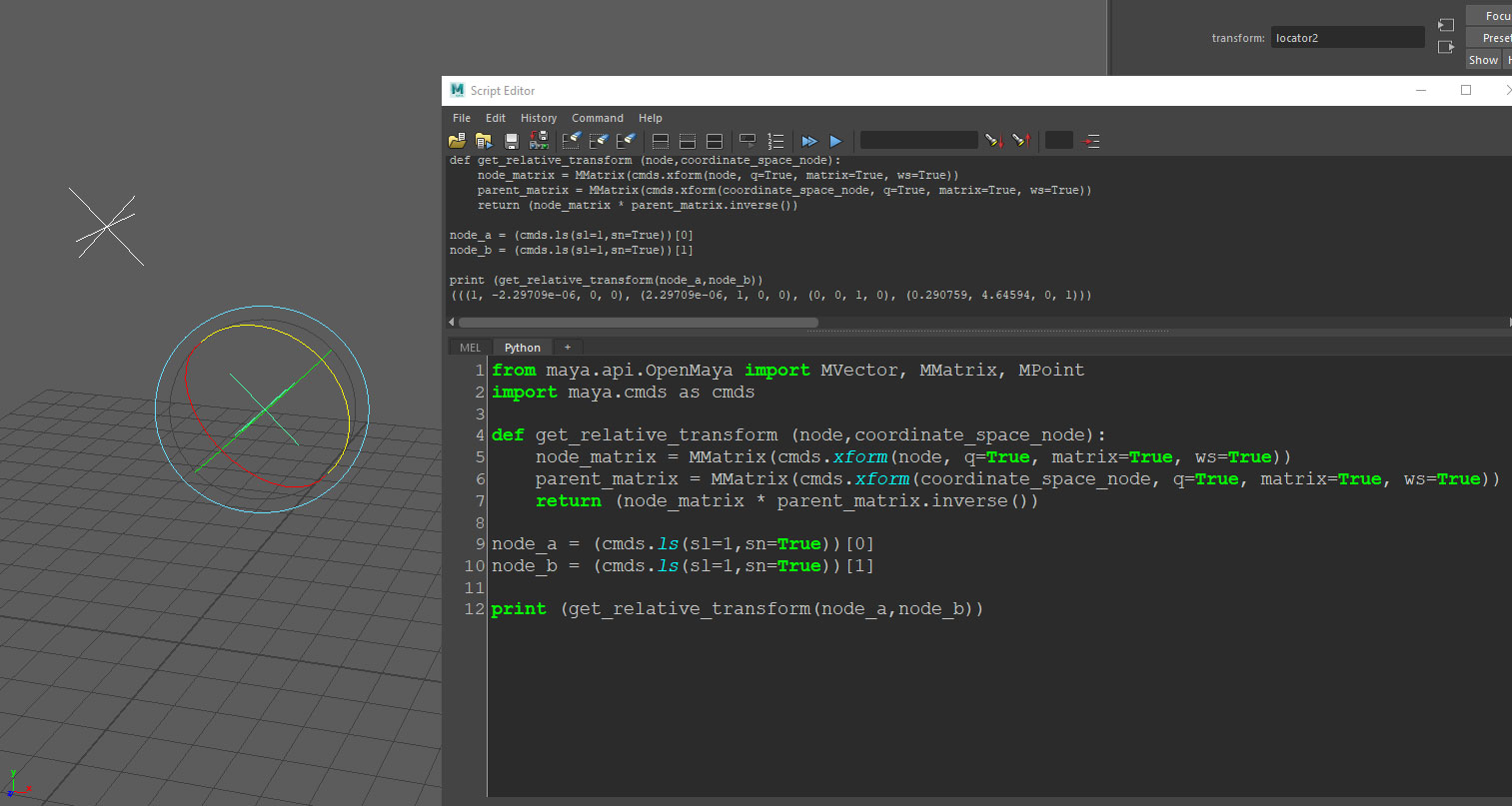# Maya Python scripting – Getting an object’s transform matrix relative to another object’s coordinates

Software:
Maya 2018

How to get an object transformation matrix relative to another object’s coordinates:
* The following script requires selecting 2 objects, the function will return the transform matrix of the first object relative to the transform matrix of the second.

```from maya.api.OpenMaya import MVector, MMatrix, MPoint
import maya.cmds as cmds

def get_relative_transform (node,coordinate_space_node):
node_matrix = MMatrix(cmds.xform(node, q=True, matrix=True, ws=True))
parent_matrix = MMatrix(cmds.xform(coordinate_space_node, q=True, matrix=True, ws=True))
return (node_matrix * parent_matrix.inverse())

node_a = (cmds.ls(sl=1,sn=True))
node_b = (cmds.ls(sl=1,sn=True))

print (get_relative_transform(node_a,node_b))```